# Faculty

In the meantime, see the departmental list and the Theory Wiki list.

## Primary Faculty

•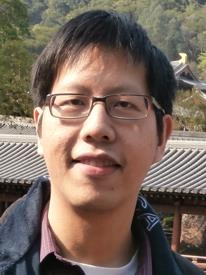Timothy Chan
Computational Geometry, Algorithms, Data Structures
•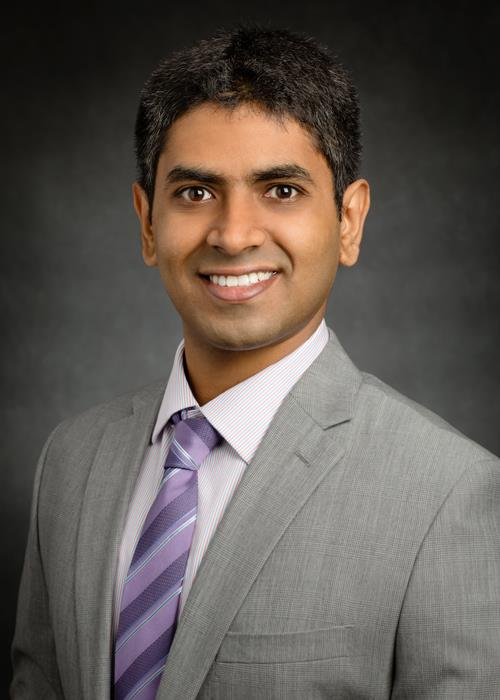Karthik Chandrasekaran
Combinatorial Optimization, Integer Programming, Probabilistic Methods and Analysis, Randomized Algorithms
•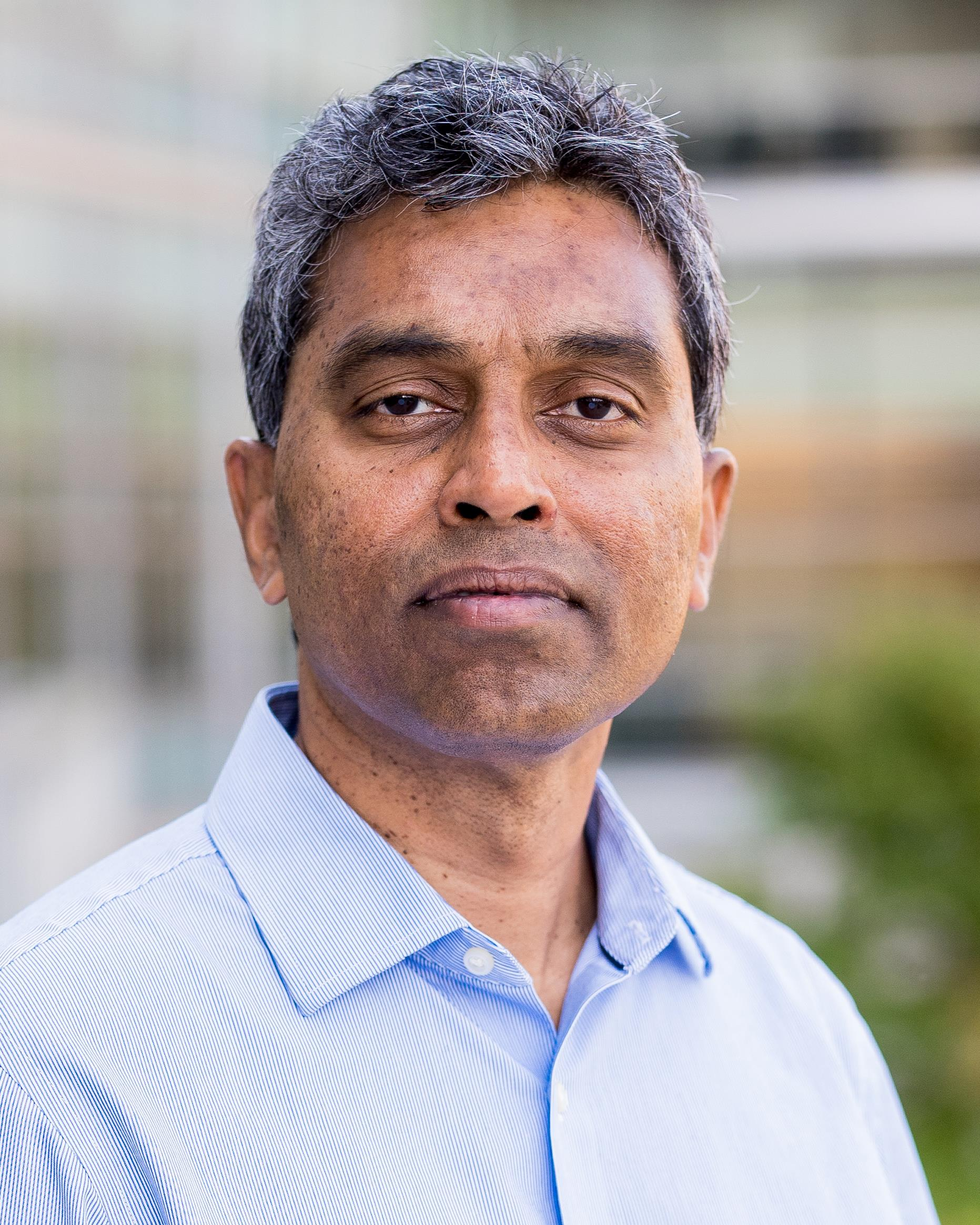Chandra Chekuri
Approximation Algorithms, Combinatorial Optimization
•Payam Delgosha
Applied Probability, Information Theory, Game Theory, Machine Learning, Quantum Information Theory
•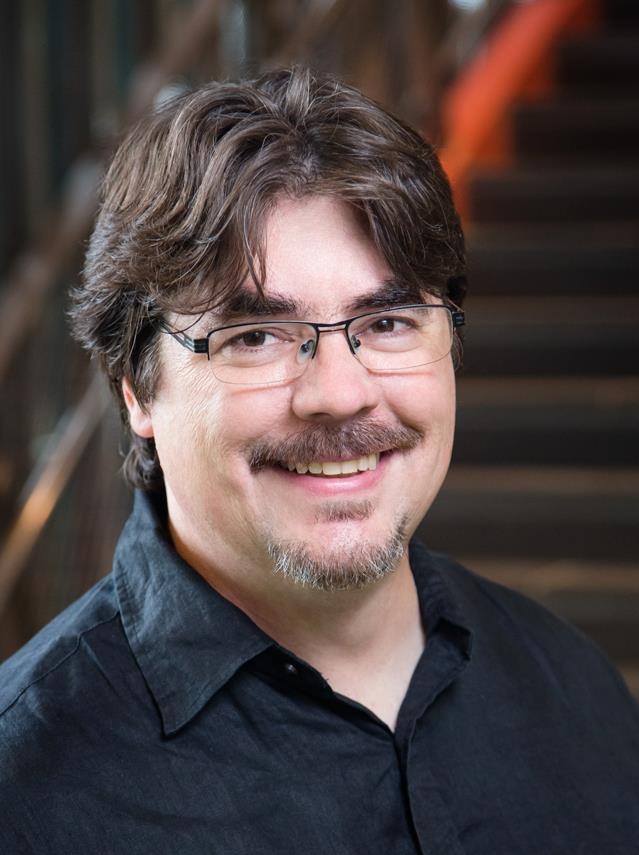Jeff Erickson
Computational Geometry and Topology, Algorithms
•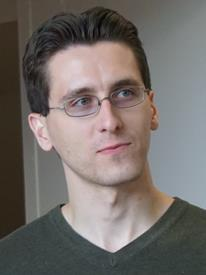Michael A. Forbes
Pseudorandomness, Algebraic Computation, Computational Complexity
•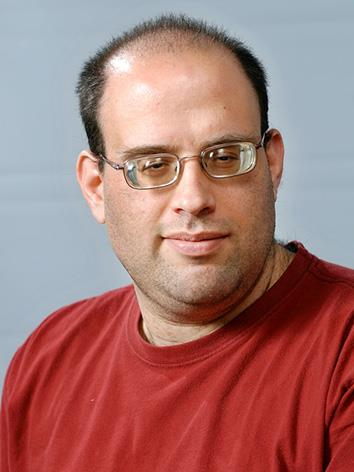Sariel Har-Peled
Computational Geometry, Geometric Approximation Algorithms
•Sheldon Jacobson
Optimization, Operations Research
•Dakshita Khurana
Cryptography, Secure Computation, Zero-Knowledge, Differential Privacy
•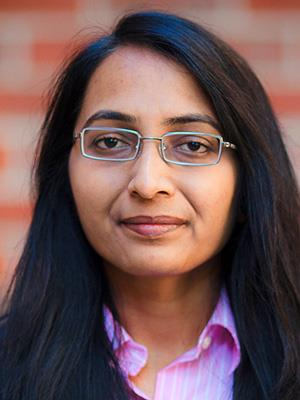Ruta Mehta
Algorithmic Game Theory, Mathematical Economics, Efficient Algorithms
•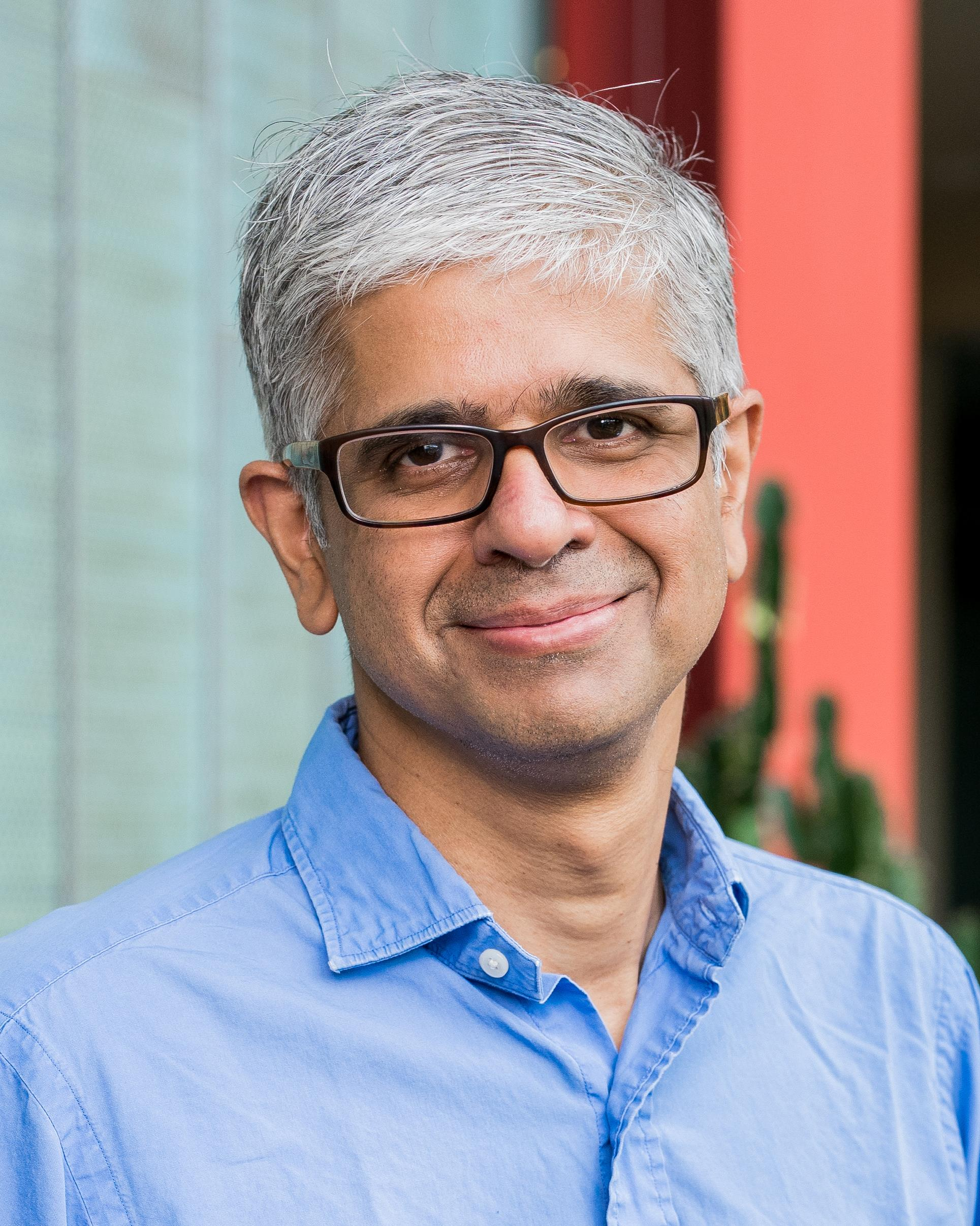Mahesh Viswanathan
Model Checking, Logic, Cyberphysical Systems, Software, Security
•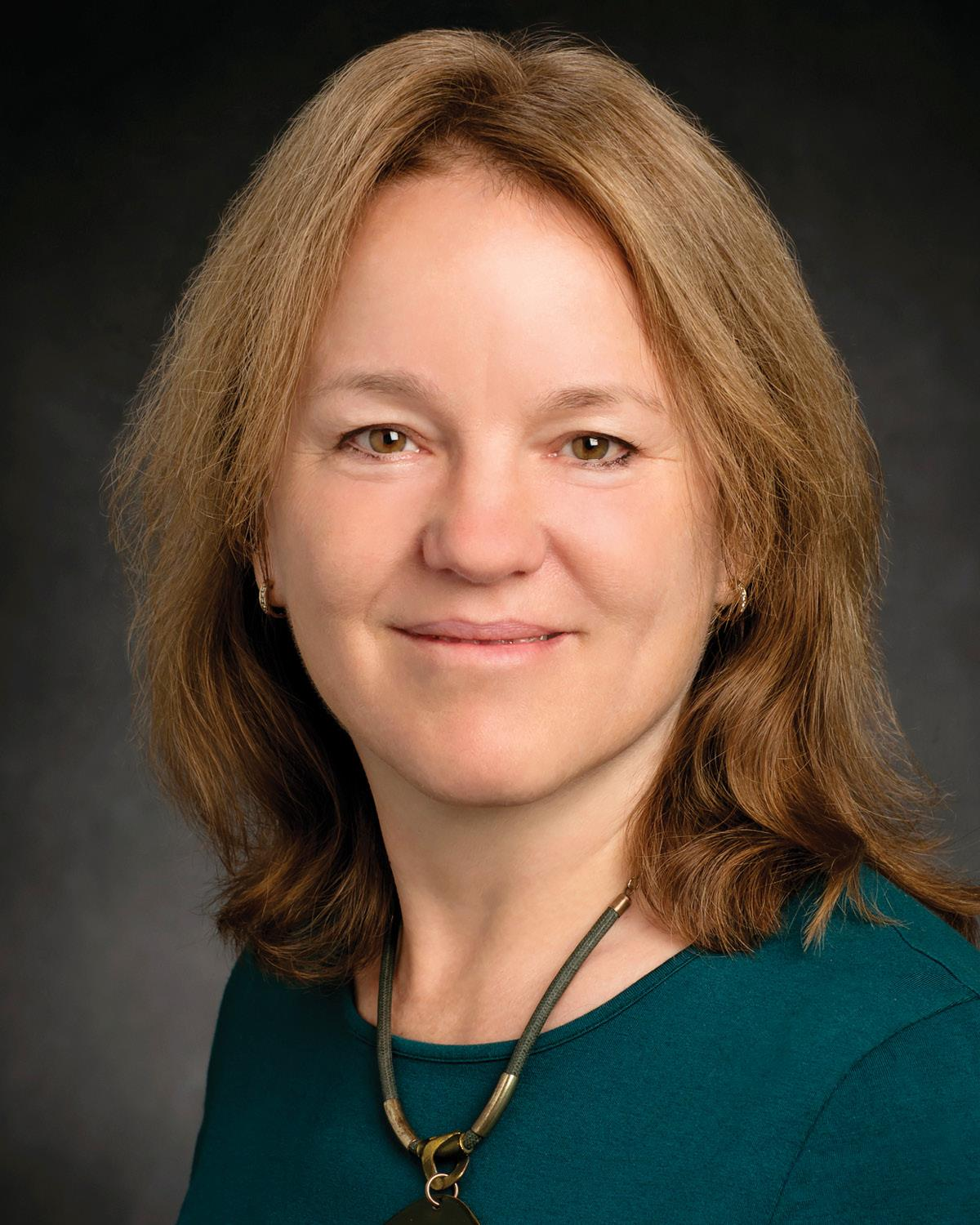Tandy Warnow
Graph Algorithms, Statistical Estimation, Heuristics for NP-Hard Optimization Problems, Experimental Algorithmics, Applications to Grand Challenges in Biology and Historical Linguistics